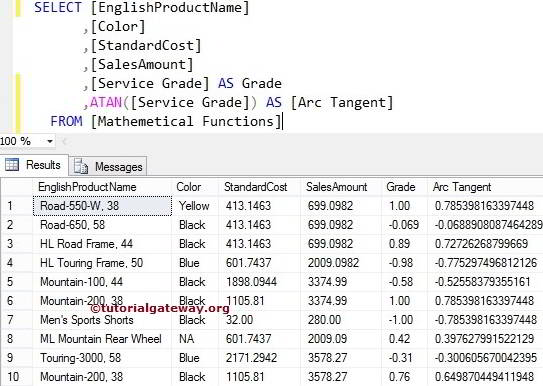# SQL ATAN Function

The SQL ATAN function is a Mathematical Function, which calculates the trigonometry Arc tangent for the specified expression. The SQL Arc tangent also called the inverse of a TANGENT Function. The syntax of the SQL Server ATAN Function is

```SELECT ATAN (Float_Expression)
FROM [Source]```

For this ATAN Function demo, we use the Math Table data

## SQL ATAN Function Example 1

The ATAN function calculates the Arctangent value for the given angle. The following query shows multiple ways to use the ATAN function.

```DECLARE @i Float
SET @i = -208.80

SELECT ATAN(@i) AS [Arc Tangent]

-- Calculating ATAN directly
SELECT ATAN(0.27) AS [Arc Tangent]

-- Calculating ATAN directly
SELECT ATAN(22.25 + 78.55 - 140.77) AS [Arc Tangent]```

We used the ATAN Mathematical Function to calculate the Arc TANGENT value for the variable @i. It means ATAN(-208.80)), and assigned a new name ‘Arc Tangent’ using ALIAS.

`SELECT TAN(@i) AS [SQL Tangent]`

## ATAN Function Example 2

We are going to calculate the Arctangent value for all the records present in [Service Grade] using this SQL Serve Function

```SELECT [EnglishProductName]
,[Color]
,[StandardCost]
,[SalesAmount]